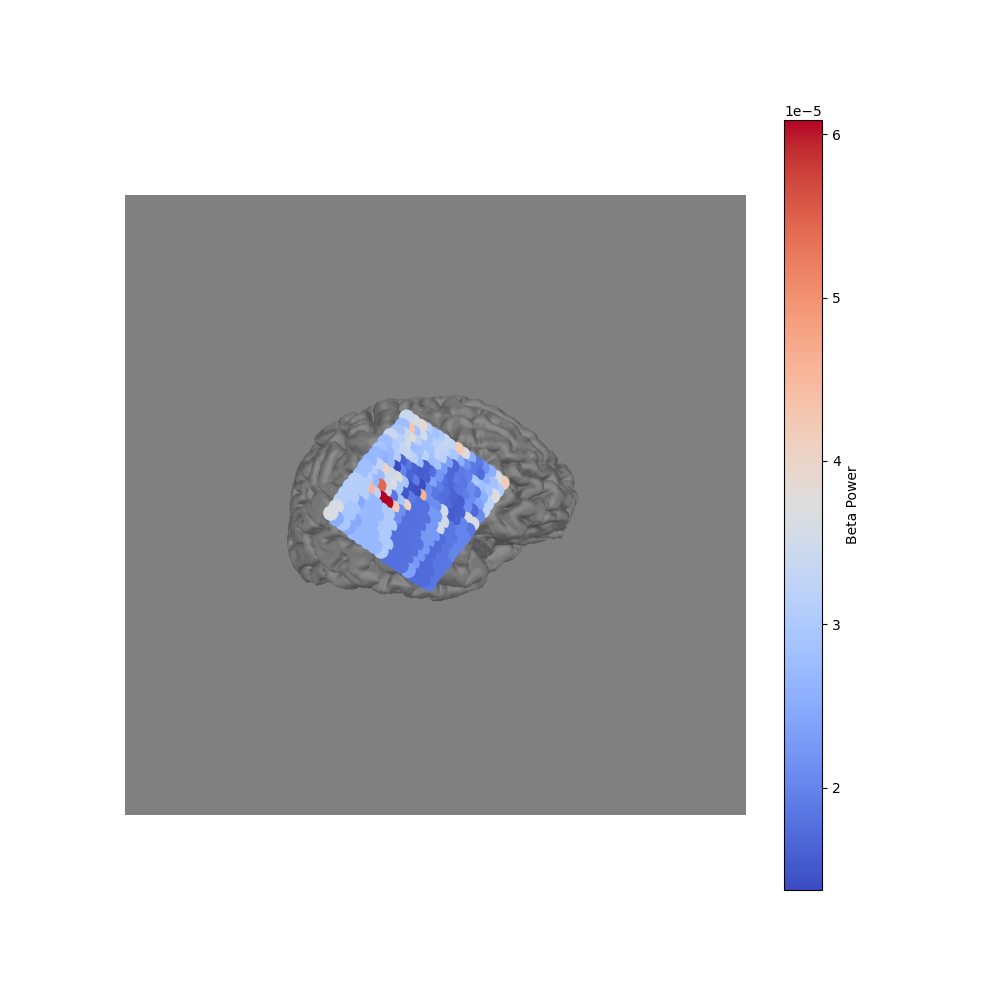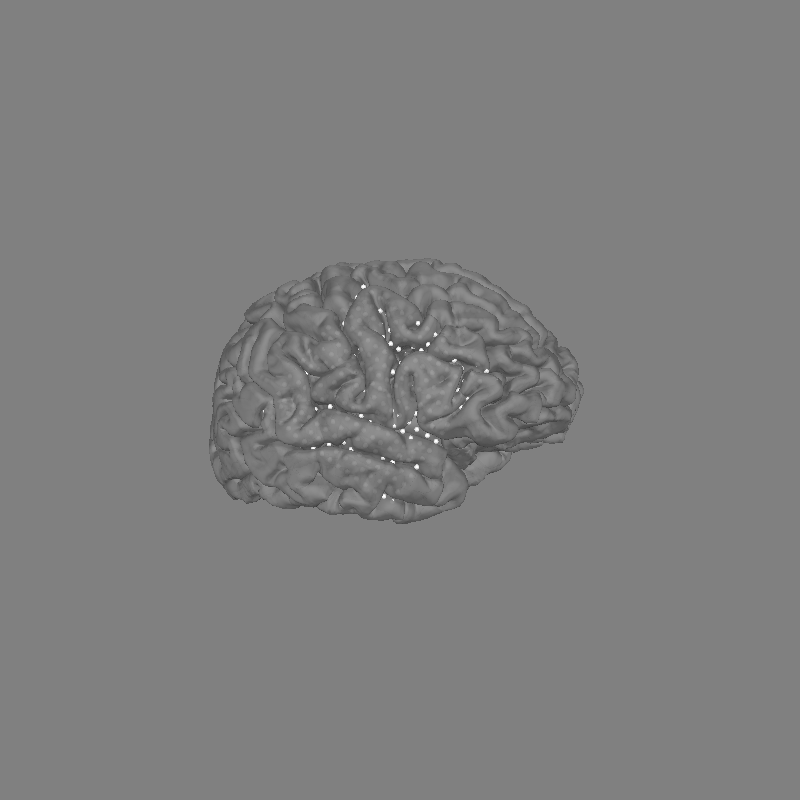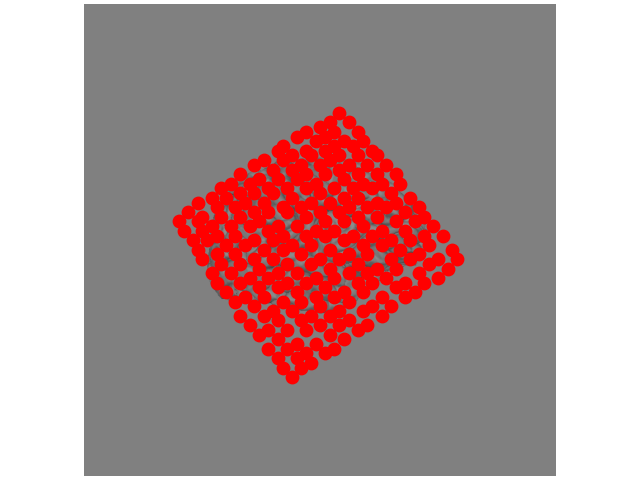# How to convert 3D electrode positions to a 2D image#

Sometimes we want to convert a 3D representation of electrodes into a 2D image. For example, if we are using electrocorticography it is common to create scatterplots on top of a brain, with each point representing an electrode.

In this example, we’ll show two ways of doing this in MNE-Python. First, if we have the 3D locations of each electrode then we can use PyVista to take a snapshot of a view of the brain. If we do not have these 3D locations, and only have a 2D image of the electrodes on the brain, we can use the `mne.viz.ClickableImage` class to choose our own electrode positions on the image.

```# Authors: Christopher Holdgraf <choldgraf@berkeley.edu>
#          Alex Rockhill        <aprockhill@mailbox.org>
#
```
```from os.path import dirname
from pathlib import Path

import numpy as np
from matplotlib import pyplot as plt

import mne
from mne.viz import ClickableImage  # noqa: F401
from mne.viz import plot_alignment, set_3d_view, snapshot_brain_montage

misc_path = mne.datasets.misc.data_path()
subjects_dir = misc_path / "ecog"
ecog_data_fname = subjects_dir / "sample_ecog_ieeg.fif"

# We've already clicked and exported
layout_path = Path(dirname(mne.__file__)) / "data" / "image"
layout_name = "custom_layout.lout"
```

First we will load a sample ECoG dataset which we’ll use for generating a 2D snapshot.

```raw = read_raw_fif(ecog_data_fname)
raw.pick_channels([f"G{i}" for i in range(1, 257)])  # pick just one grid

# Since we loaded in the ecog data from FIF, the coordinates
# are in 'head' space, but we actually want them in 'mri' space.
# So we will apply the head->mri transform that was used when
# generating the dataset (the estimated head->mri transform).
montage = raw.get_montage()
montage.apply_trans(trans)
```
```Opening raw data file /home/circleci/mne_data/MNE-misc-data/ecog/sample_ecog_ieeg.fif...
Range : 0 ... 112 =      0.000 ...     0.700 secs
NOTE: pick_channels() is a legacy function. New code should use inst.pick(...).
```

## Project 3D electrodes to a 2D snapshot#

Because we have the 3D location of each electrode, we can use the `mne.viz.snapshot_brain_montage()` function to return a 2D image along with the electrode positions on that image. We use this in conjunction with `mne.viz.plot_alignment()`, which visualizes electrode positions.

```fig = plot_alignment(
raw.info,
trans=trans,
subject="sample_ecog",
subjects_dir=subjects_dir,
surfaces=dict(pial=0.9),
)
set_3d_view(figure=fig, azimuth=20, elevation=80)
xy, im = snapshot_brain_montage(fig, montage)

# Convert from a dictionary to array to plot
xy_pts = np.vstack([xy[ch] for ch in raw.ch_names])

# Compute beta power to visualize
beta_power = raw.filter(20, 30).apply_hilbert(envelope=True).get_data()
beta_power = beta_power.max(axis=1)  # take maximum over time

# This allows us to use matplotlib to create arbitrary 2d scatterplots
fig2, ax = plt.subplots(figsize=(10, 10))
ax.imshow(im)
cmap = ax.scatter(*xy_pts.T, c=beta_power, s=100, cmap="coolwarm")
cbar = fig2.colorbar(cmap)
cbar.ax.set_ylabel("Beta Power")
ax.set_axis_off()

# fig2.savefig('./brain.png', bbox_inches='tight')  # For ClickableImage
``````Channel types:: ecog: 256
Reading 0 ... 112  =      0.000 ...     0.700 secs...
Filtering raw data in 1 contiguous segment
Setting up band-pass filter from 20 - 30 Hz

FIR filter parameters
---------------------
Designing a one-pass, zero-phase, non-causal bandpass filter:
- Windowed time-domain design (firwin) method
- Hamming window with 0.0194 passband ripple and 53 dB stopband attenuation
- Lower passband edge: 20.00
- Lower transition bandwidth: 5.00 Hz (-6 dB cutoff frequency: 17.50 Hz)
- Upper passband edge: 30.00 Hz
- Upper transition bandwidth: 7.50 Hz (-6 dB cutoff frequency: 33.75 Hz)
- Filter length: 107 samples (0.669 s)

[Parallel(n_jobs=1)]: Done  17 tasks      | elapsed:    0.0s
[Parallel(n_jobs=1)]: Done  71 tasks      | elapsed:    0.0s
[Parallel(n_jobs=1)]: Done 161 tasks      | elapsed:    0.0s
```

## Manually creating 2D electrode positions#

If we don’t have the 3D electrode positions then we can still create a 2D representation of the electrodes. Assuming that you can see the electrodes on the 2D image, we can use `mne.viz.ClickableImage` to open the image interactively. You can click points on the image and the x/y coordinate will be stored.

We’ll open an image file, then use ClickableImage to return 2D locations of mouse clicks (or load a file already created). Then, we’ll return these xy positions as a layout for use with plotting topo maps.

```# This code opens the image so you can click on it. Commented out
# because we've stored the clicks as a layout file already.

# # The click coordinates are stored as a list of tuples
# click = ClickableImage(im)
# click.plot_clicks()

# # Generate a layout from our clicks and normalize by the image
# print('Generating and saving layout...')
# lt = click.to_layout()
# lt.save(layout_path / layout_name)  # save if we want

lt = mne.channels.read_layout(layout_path / layout_name, scale=False)
x = lt.pos[:, 0] * float(im.shape)
y = (1 - lt.pos[:, 1]) * float(im.shape)  # Flip the y-position
fig, ax = plt.subplots()
ax.imshow(im)
ax.scatter(x, y, s=80, color="r")
fig.tight_layout()
ax.set_axis_off()
```Total running time of the script: (0 minutes 10.441 seconds)

Estimated memory usage: 25 MB

Gallery generated by Sphinx-Gallery